Next: 4.2 Interface Models Up: 4 Description of the Simulator Previous: 4 Description of the Simulator

4.1 Volume Models

In all semiconductor segments Poisson's equation and the continuity equation for each simulated carrier type have to be solved. Poisson's equation, derived from the third Maxwell equation, reads: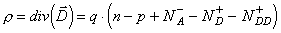, (16)

where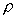is the total electric charge density,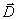denotes the displacement vector, q is the electronic charge, n and p are the electron and hole concentrations,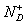and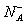are the concentrations of ionized donors and acceptors, and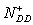is the concentration of ionized deep traps.

The continuity equation for electrons and holes can be derived taking the zero order momentum of the Boltzmann equation [44, 45]: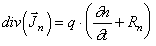(17)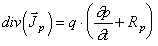. (18)

Taking the first order momentum, the Boltzmann equation yields the current densities for electron and holes: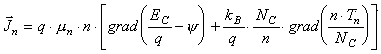(19)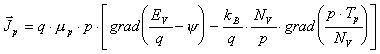. (20)
Here, NC and NV are the effective densities of states in the conduction and valence band respectively, EC and EV denote the conduction and the valence band edges, and µn and µp are the mobilities of electrons and holes, respectively. These parameters account for different materials. kB is the Boltzmann constant. If the temperature Tn of electrons and Tp of holes are constant, i. e. equal to the lattice temperature, (19) and (20) reduce to the equations for drift diffusion. For a hydrodynamic model Tn and Tp have to be taken into account. In this case the energy transport equations obtained from the second order momentum of the Boltzmann equation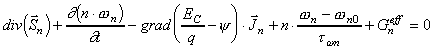(21)

and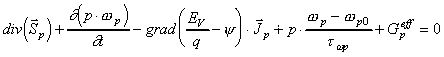(22)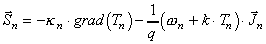(23)

and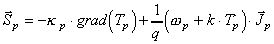(24)

are the energy fluxes for electrons and holes,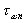andare the energy relaxation times, and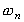and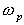are the energy of electrons and holes, respectively . At room temperature the drift kinetic energy of the carriers is relatively low compared to the random kinetic energy of the carriers and can be neglected. Therefore the approximation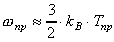(25)

for the carrier energy can be used. According to the Wiedemann­Franz law the thermal conductivities of electrons and holes are calculated by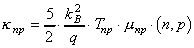. (26)

Helmut Brech
1998-03-11